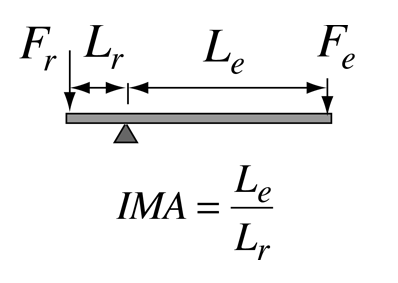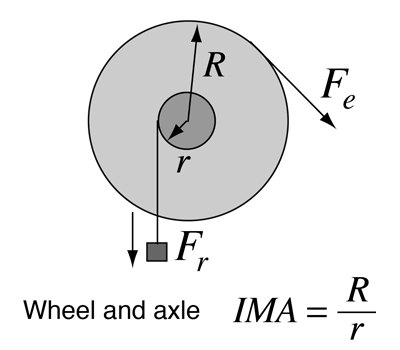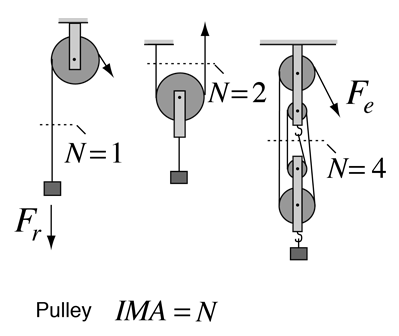# The LeverThe lever is one of the so-called "simple machines" from which many more complex machines are derived. With a lever, one can obtain a multiplication of force, but of course not a multiplication of energy. The multiplication of force can be seen to arise from the equilibrium of torques, where an input force Fe with a long lever arm Le can balance a larger resistance force Fr with a short lever arm Lr.

A rigid lever can approach an ideal machine since there is very little loss. From torque equilibrium we see that a resistance force Fr can be balanced by a smaller effort force Fe = (Lr/Le)Fr. This is often stated in terms of the ideal mechanical advantage Fr/Fe = Le/Lr shown in the illustration.

Since we know by conservation of energy that no machine can output more energy than was put into it, the ideal case is represented by a machine in which the output energy is equal to the input energy. For simple geometries in which the forces are in the direction of the motion, we can characterize the ideal machine in terms of the work done as follows:

Ideal Machine: Energy input = Energy output

Work input = Fedinput = Frdoutput = Work output

From this perspective it becomes evident that a simple machine may multiply force. That is, a small input force can accomplish a task requiring a large output force. But the constraint is that the small input force must be exerted through a larger distance so that the work input is equal to the work output. You are trading a small force acting through a large distance for a large force acting through a small distance. This is the nature of all the simple machines above as they are shown.

Of course it is also possible to trade a large input force through a small distance for a small output force acting through a large distance. This is also useful if what you want to achieve is a higher velocity. Many machines operate in this way.

 Simple Machines
Index

Torque Concepts

 HyperPhysics***** Mechanics R Nave
Go Back

# Wheel and AxleThe wheel and axle combination constitutes one of the so-called "simple machines" from which many more complex machines are derived. The principle of operation is essentially a lever, since it depends upon the effort force Fe having a longer lever arm than the resistance force Fr The ideal mechanical advantage is just the ratio of those lever arms R/r.

The clear advantage of the wheel and axle over a simple lever is that the distance of travel is limited only by the amount of rope or cable that you can wrap around the wheel or axle.

 Simple Machines
Index

Torque Concepts

 HyperPhysics***** Mechanics R Nave
Go Back

# The PulleyThe pulley is one of the so-called "simple machines" from which many more complex machines are derived. With a single fixed-axis pulley, the ideal mechanical advantage is just N=1. You get the convenience of being able to redirect the effort force Fe, so that you can stand clear of the load. With a suspended pulley as in the middle illustration, the upward forces in the two ropes is equal, and therefore each supports half of the load, giving an IMA of N=2.

With a four-pulley set as shown, you have four ropes supporting the load, so the effort force Fe that establishes the rope tension is just one-fourth of the load in the ideal case, so IMA=4. All these force relationships are obtained from the force equilibrium condition, which in this case just amounts to "forces up = forces down" at any cross-section of the system.

 Simple Machines
Index

Torque Concepts

 HyperPhysics***** Mechanics R Nave
Go Back